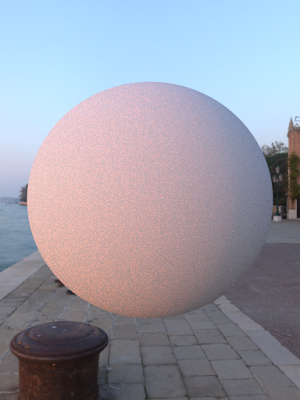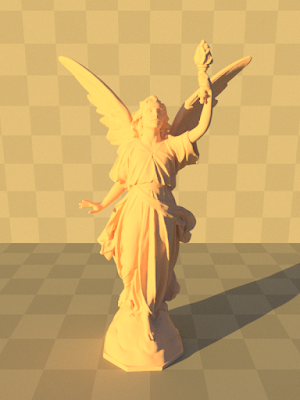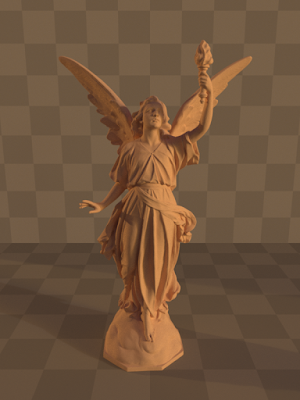### Image Based Lighting

Although it is possible to mimic real world lighting with various area light sources and analytical skylights to some extent, it is pretty hard to achieve what can be represented by image based lights (environment lights). Using an image based light source adds a great level of realism to scenes without having to create an entire environment just for lighting purposes. Because images of these light sources are stored as one of the hdr file formats, high dynamic range of scenes is well preserved so that the correct radiance distribution can be used.

Image based lights should not be distinguished from other light sources in order to keep a generic light interface. So, an integrator must be able to do the followings at some surface:
• Sample a direction according to the pdf of this light source.
• Get pdf value for a certain direction, that is, probability of choosing this direction according to the pdf of this light source.
• Get radiance value for a certain direction, that is, how much radiance exits from this light source through this direction.
For diffuse area lights, it is rather easy to construct a pdf so that each point on the area is selected with the same probability. However, since environment lights are represented by images, uniform random selection among pixels might cause a high variance since radiance values among pixels might vary significantly. Therefore, special care must be taken when constructing a pdf for an image based light. As it is explained in Physically Based Rendering: From Theory to Implementation, pdf value of a particular pixel can be proportional to the luminance value of that pixel, that is, $p(i, j) \propto I(i, j)_{Luminance}$. If entire cdf is computed in both dimensions of the image, a 2D discrete sampling mechanism might do the trick. A column of the image is selected first and then a row is selected from this particular column or vice versa. After selecting position $(i, j)$ on the image, this tuple should be converted to $(u, v)$ and then to a direction $(x, y, z)$ in order to provide a sampled direction to the integrator. Given a direction, pdf or radiance value for this direction can be easily computed by converting back to $(i, j)$.

The most important part is to provide a pdf value in solid angle domain since the integrator works in this domain while computing the rendering equation. So, when giving a pdf value for a direction to the integrator, a correct pdf transformation should be applied. In this case, $p(i, j)$ should be transformed to $p(w)$. We can use series of transformation to achieve this. We know that $p(\theta, \phi) = p(w) sin(\theta)$. Also by computing the determinant of the jacobians of the related transformations, we find that $p(\theta, \phi) 2\pi^2 = p(u, v)$ and $p(u, v) = p(i, j) \cdot w \cdot h$ where $w$ and $h$ are the width and height of the image, respectively. Thus, we have $p(w) = \frac{p(i, j) \cdot w \cdot h}{2\pi^2sin(\theta)}$.

While implementing an environment light, a sphere with infinite (practical infinity) radius can be placed into the origin of the world coordinate system, $(0, 0, 0)$. However, this is not really necessary since direction of a ray that do not intersect with anything can be converted from $(x, y, z)$ to $(i, j)$ to get the radiance value from the light source. Glue uses latter approach and treats environment light in a generic way so that no special treatment in the integrator has to be made for it.

Relevant implementation is:
Importance of constructing a pdf based on luminance values can be seen below. First image lacks reddish part of light which has highest luminance values. Second image uses a pdf explained in this post and captures the reddish values. Third image shows that if multiple importance sampling is used so that the cosine term is also used for sampling, variance reduces fairly in the right side of the sphere.123
Some images rendered in different environments:SunriseMoonlightSunsetNight - Street lamps
Also, some extra images:Diffuse material: Kd controlled by perlin textureGold: Roughness controlled by UV texture